# Python基础知识学习(十)——数值与日期：数值、随机数、日期的一些操作685-杜同学

## 热门标签

### 1.数值

• ’{}‘.format(a)或 f'{a}' —— 打印a
• '{:f}'.foramat(a)或f'{a:f}'  —— 以浮点型打印a
• '{:+f}'.foramat(a)或f'{a:+f}'  —— 带加号的浮点型a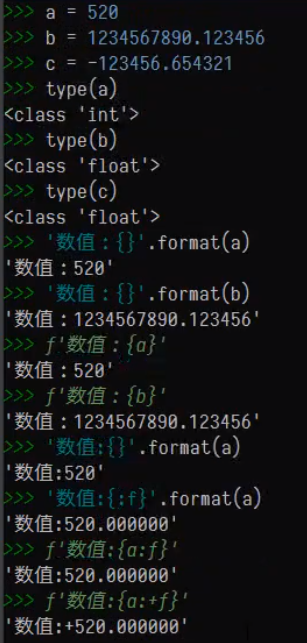• '{:,f}'.foramat(a)或f'{a:,f}' —— 千分位,间隔a
• '{:,.4f}'.foramat(a)或f'{a:,.4f}' —— 以千分位间隔并保留小数点后四位（四舍五入）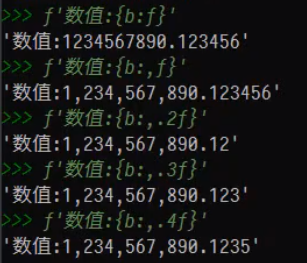• '{:.2%}'.foramat(a)或f'{a:.2%}' —— 将a打印成带百分号并保留两位小数的形式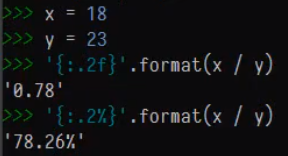• math.trunc(数值) —— 截断
• math.floor(数值) —— 向下取整
• math.ceil(数值) —— 向上取整

• round(数值 ，小数位数默认=0 ) —— 四舍五入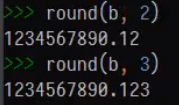### 2.随机数

• random.choice(l) 序列中随机选择一个值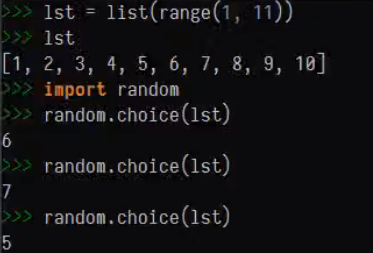• random.sample(l，3) 获取指定数目的序列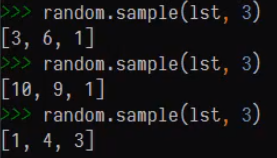• random.shuffle(l) 打乱顺序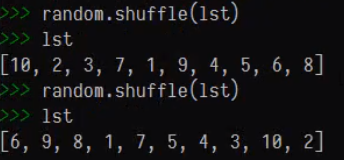• random,randint(1,10) 生范围内的随机整数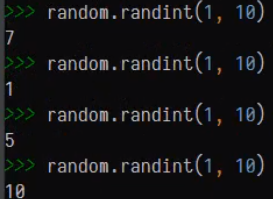• random.random() 生成0-1的随机浮点数• random.getrandbits() 生成指定bit位数的随机数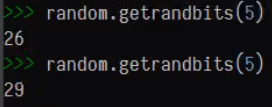• 总结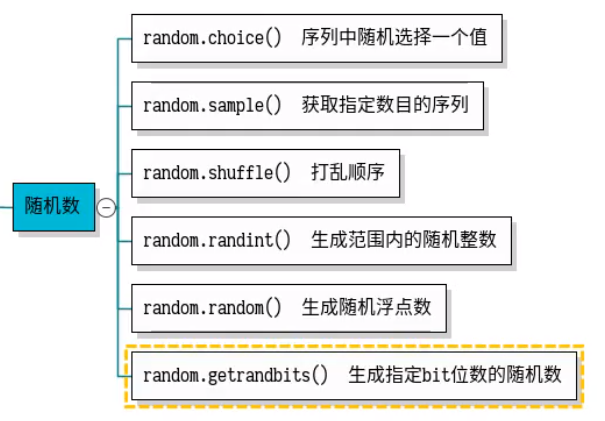### 3.日期

import datetime

• datetime.date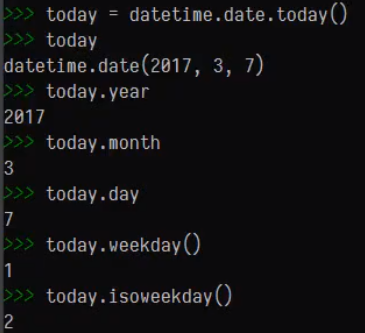• datetime.time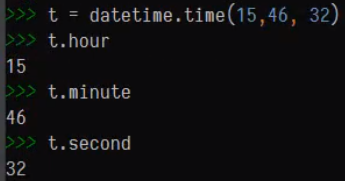• datetime.datetime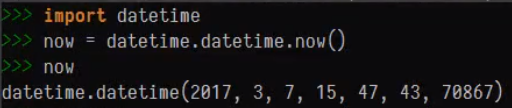• 字符串与日期的格式转换 —— datetime.datetime.strptime()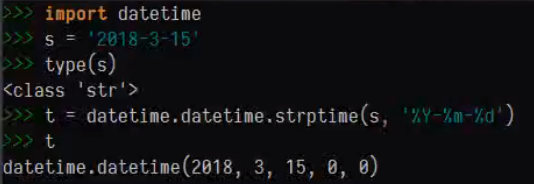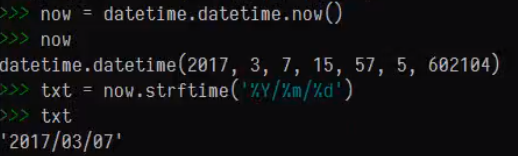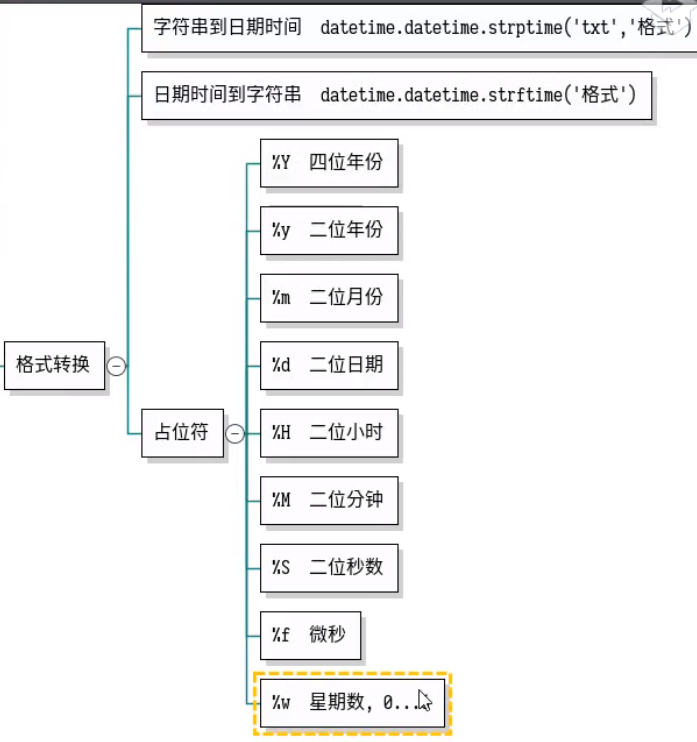•  时间差 datetime.timedelta()

days = 100 —— 加100天

hours = -100 —— 减100小时

seconds = 100 —— 加100秒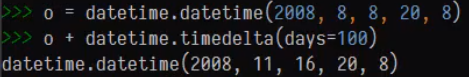### 评论 抢沙发### 觉得文章有用就打赏一下文章作者

#### 支付宝扫一扫打赏#### 微信扫一扫打赏Vieu3.3主题Q Q 登 录